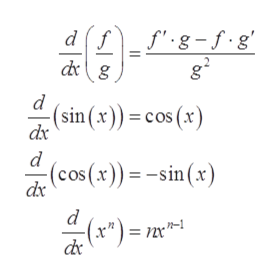# -11xLet f(x)Evaluate f( at nsin(r)cos(r)

Question

Evaluate f′(x) at x = π.

check_circleExpert Solution
Step 1

To determine the value of f’(x) at x = π.

Step 2

Given:

Step 3

Formula Used:

...help_outlineImage Transcriptionclosefg-f.g df dg d (sin (x))= cos (x) dx (cos(x)) =-sin (x) dx e") = 2x fullscreen

### Want to see the full answer?

See Solution

#### Want to see this answer and more?

Solutions are written by subject experts who are available 24/7. Questions are typically answered within 1 hour*

See Solution
*Response times may vary by subject and question
Tagged in

### Derivative